# PSAT Math : Statistics

## Example Questions

### Example Question #3 : How To Find Mode

What is the mode of the following set of numbers?

24, 38, 26, 27, 7, 27, 34, 12, 13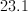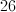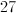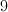Explanation:

The mode is the number that appears the most in a given set of numbers.

### Example Question #2 : Mode

Consider the following dataset: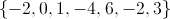The arithmetic mean of the dataset is.

The median of the dataset is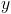.

The range of the dataset is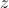.

The mode of the dataset is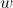.

Which of the following statements is true?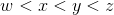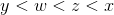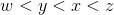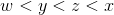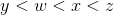Explanation:

In general: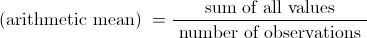In this case the mean is: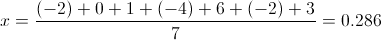To obtain the median, arrange the observations from smallest to largest: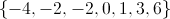When there is an odd number of observations, the median is the middle value. In this case: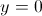The range is the difference between the largest and smallest value: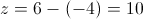The mode is the value that occurs most often in the dataset: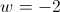Since: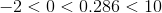we can conclude:### Example Question #1 : How To Find Mode

 Marker Colors Students Blue 13 Pink 10 Orange 5 Brown 5 Green 7

The above chart shows the number of students in a class who chose each of the five marker colors available.

What is the mode of the above chart?Explanation:

The mode of a problem is the most common number, i.e. the number that appears the most times in a given set of data. In this case, only one number is repeated. Therefore, the mode is 5.

### Example Question #2 : How To Find Mode

Find the mode(s) of the following set of numbers: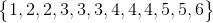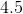andandandandExplanation:

The mode is the most frequently represented number in a data set. In this instance, that isand. There can be more than one mode.

### Example Question #2 : How To Find Mode

Find the mode of the following set of numbers: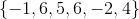Explanation:

The mode is the most frequently occurring number in a data set. In this instance, that is.

### Example Question #81 : Statistics

Find the range of the following sequence:

1, 3, 4, 6, 11, 23

5

22

6

8

22

Explanation:

To find the range, subtract the smallest number from the largest number. In this case we get 23 - 1 = 22 so the range is 22.

### Example Question #1 : Range

What is the range of the data set below?

103, 132, 241, 251, 623, 174, 132, 170, 843, 375, 120, 641, 384, 222, 833

833

740

103

545

473

740

Explanation:

The range of a set of data is found by subtracting the smallest item from the largest.  In this case, 843 - 103 = 740.

### Example Question #1 : Range

Find the range of the data set:

25, 83 , 51, 13, 37, 21, 52, 58

42.5

70

83

44

13

70

Explanation:

44 is the median of the data.  42.5 is the arithmetic mean.  13 is the minimum value.  83 is the maximum value.

To find the range, subtract the minimum value from the maximum value:

83 - 13 = 70

### Example Question #1 : How To Find Range

F, G, H are the only three numbers in a sequence in which each number is twice the number before it. The three number have an arithmetic mean of 21. What is the value of H?

36

22

63

30

24

36

Explanation:

We know that H = 2G = 4F, and G = 2F and that (F + G + H)/3=21. If we substitute each term with its F equivalent we get:

(F + 2F + 4F)/3 = 21

7F/3 = 21

7F = 63

F = 9

If we substitute F = 9 into H = 4F, we get H = 36.

### Example Question #3 : How To Find Range

A set of numbers consists of eleven consecutive even integers. If the median of the set is 62, what is the range of the set?

10
52
20
40
62
Explanation:

We don't know what the numbers are yet, so let's just call them a1, a2, a3, a4....a11. Let's assume a1 is the smallest, and a11 is the greatest.

If we were going to find the median of our set, we would need to line them all up, from least to greatest.

a1, a2, a3, a4, a5, a6, a7, a8, a9, a10, a11

The median is the middle number, which in this case about be a6, because there are five numbers before it and five numbers after it.

We know that the median is 62, so that means that there must be five numbers before 62 and five numbers after. Because the numbers are all consecutive even integers, the set must look like this:

52, 54, 56, 58, 60, 62, 64, 66, 68, 70, 72

To find the range of this set, we must find the difference between the largest and smallest numbers. The largest is 72, and the smallest is 52, so the range is 72 - 52 = 20.